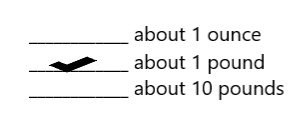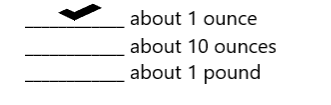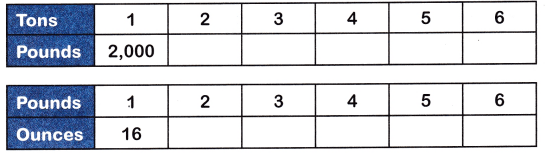# McGraw Hill Math Grade 4 Chapter 11 Lesson 2 Answer Key Customary Units of Weight

Practice the questions of McGraw Hill Math Grade 4 Answer Key PDF Chapter 11 Lesson 2 Customary Units of Weight to secure good marks & knowledge in the exams.

## McGraw-Hill Math Grade 4 Answer Key Chapter 11 Lesson 2 Customary Units of Weight

Solve

Place a check mark next to the best answer.

Question 1.
What does a baby rabbit weigh?Explanation:
A baby rabbit weigh about 1 pound.

Question 2.
What does a cherry weigh?Explanation:
A cherry weigh about 1 ounce.

Question 3.
What does a gallon jug of milk weigh?Explanation:
A gallon jug of milk weigh about 9 pounds.

Question 4.
Multiply to complete the tables.